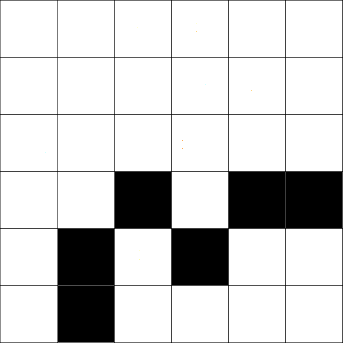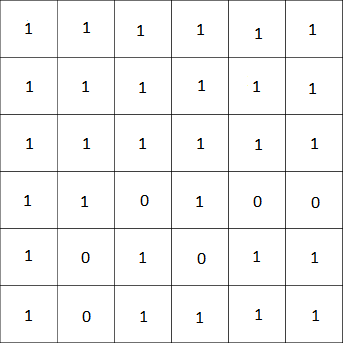## Introduction

Prerequisites: Depth First Search, Breadth First Search

Flood fill is a search that starts at a point and finds the areas connected to the start point. For example, the "bucket fill" in Photoshop or MS Paint uses flood fill to fill in the connecting areas of the same colour.

Flood fill can be implemented using a BFS or DFS.The above grid shows how flood fill works. The number in each cell represents the number of steps from the starting cell and the black cells are blocked off. We start at the cell marked as 0 and we reach all adjacent cells.

## Bucket Fill

Given an N x M matrix and a start point and two colors (src and dst), we want to replace all the cells in the matrix that are connected to the start point with the color src and change to color dst as well as output the number of cells changed.

Example:We use bucket fill in the top left corner of the bitmap to fill everything with the same color as the cell.A numeric representation of the bitmap before bucket fill:Numeric representation of the bitmap after bucket fill in the left:### DFS Solution

This is the DFS approach to the problem.

• Assume that n,m are global integers that are the width and height of the image
• Assume that image is a global integer n by m matrix for the image
• Assume that visited is a global boolean n by m matrix that is initially all false
```public int FloodFillDFS(int x, int y, int src, int tar) {
if (x < 0 || x >= n || y < 0 || y >= m) {
return 0;
}
if (visited[x][y]) {
return 0;
}
visited[x][y] = true;
if (image[x][y] != src) {
return 0;
}
image[x][y] = tar;
int sum = 0;
sum += FloodFillDFS(x + 1, y, src, tar);
sum += FloodFillDFS(x - 1, y, src, tar);
sum += FloodFillDFS(x, y + 1, src, tar);
sum += FloodFillDFS(x, y - 1, src, tar);
return sum;
}
```

### BFS Solution

This is the BFS approach to the problem.

• Assume that n,m are global integers that are the width and height of the image
• Assume that image is a global integer n by m matrix for the image
• Assume that visited is a global boolean n by m matrix that is initially all false
```public int FloodFillBFS(int x, int y, int src, int tar) {
q.push(new Point(x, y));
int total = 0;
while (q.isEmpty() == false) {
Point cur = q.pop();
if (cur.x < 0 || cur.x >= n || cur.y < 0 || cur.y >= m) {
continue;
}
if (visited[cur.x][cur.y]) {
continue;
}
visited[cur.x][cur.y] = true;
if (image[cur.x][cur.y] != src) {
continue;
}
image[cur.x][cur.y] = tar;
total++;
q.push(new Point(cur.x + 1, cur.y));
q.push(new Point(cur.x - 1, cur.y));
q.push(new Point(cur.x, cur.y + 1));
q.push(new Point(cur.x, cur.y - 1));
}
}
```

## Exercises

1. Given a NxN grid and a list of starting cells and wall cells, find the distance to the closest start point for every non-wall grid space without passing through a wall.
2. Given a grid of numbers where 0 means empty space and 1 means a wall, find the number of rooms and the perimeter of each room. The border of the grid is guaranteed to be walls.
```1 1 1 1 1 1
1 0 0 0 1 1
1 1 0 1 1 1
1 1 0 1 0 1
1 1 1 1 1 1
```

The number of rooms is 2, the perimeter of the first room is 12 and the perimeter of the second room is 4.